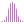#LinRegIntercept (MDX)

Calculates the linear regression of a set and returns the value of the intercept in the regression line, y = ax + b.

#### Syntax

LinRegIntercept( <List> , , )